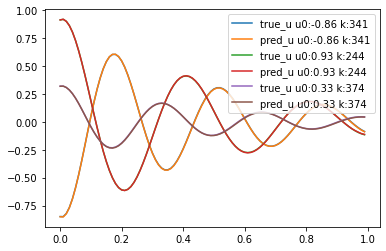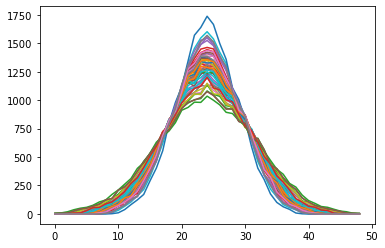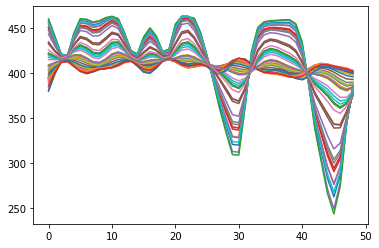# FNO fails when using Gaussian outputs (particles accelerator)

Good Morning,

We are trying to use Nvidia Modulus to accelerate the simulation of the beam in particles accelerator with neural operators. We started with a very simple problem of FNO (and not related with particles accelerator), a dumped armonic oscillator where we change the initial displacement u0 and elastic constant k, and we simulate in time domain window to obtain de response. As the discretization of inputs and outputs has to be the same, we stablished the the inputs u0 an k as constant vectors through time. With this very simple example we had good results that you can see in the next image:Now we try to train the FNO to simulate the particle distribution in a particle accelerator after a series of magnets (which change the shape of the beam). In this very simple example, we have only 3 magnets with 1 parameter each one. We change this 3 parameters ‘k’ and that changes the shape of the particles at the end of accelerator (it’s always a Gaussian shape). We tried the same method that the oscillator example, with 3 matrices constant through 2D space as input, and the particle distribution shape as an output (this is only 2D in space, there is not time domain).

The true output for example would be:

The problem is that the model doesn’t learn, we see ‘bands’ as outputs (and for us is a very similar, even simpler case than Darcy Flow FNO example) and the loss fluctuates. For example:

We tried to simplify the problem to 1 dimension, and trying to predict de spatial distribution in only one axe.
These would be the true outputs of same examples:But this is what we obtain, the same bands but in 1D:We tried to change FNO hyperparameters , batch size, learning rate, etc, but nothing seems to work. We have successfully trained the FNO for way more complex systems and we don’t see way doesn’t work with this specific example (we trained a base model MLP and works well). We suspect that the fact that the FFT of a Gaussian distribution is another Guassian distribution could be related. Could you help us clarify why the FNO could not work in this specific but simple example o what is the error we could be making ?

Thank you